SYLLABUS  Previous: 1.2 Differential Equations  Up: 1.2 Differential Equations  Next: 1.2.2 Partial differential equations

### 1.2.1 Ordinary differential equations

Slide : [ ODE Euler - mid-point rule - Runge-Kutta || VIDEO login]

Rather than repeating details of elementary numerical analysis, this section is only meant to review a few methods that will be used later, e.g. in the chapter dealing with the Monte Carlo method for partial differential equations (PDE).

Ordinary differential equations (ODE) are often encountered when dealing with initial value problems. In Newtonian mechanics, for example, the trajectory of a particle is described by an evolution in time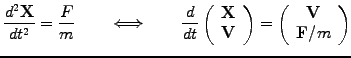The example shows how higher order equations can be recast into a system of first order equations, with components of the form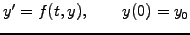(1.2.1#eq.1)

Under very general assumptions, an initial condition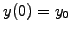having the same number of components as the unknown vector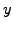yields exactly one solution to (1.2.1#eq.1), which can then be approximated with a computer.

Introducing a discretization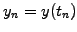with a finite number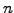of time steps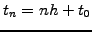, a straight forward manner to solve an ODE is to approximate the derivative with a finite difference quotient forward in time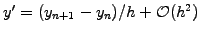, leading to the Euler recursion formula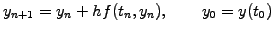(1.2.1#eq.2)

Because all the quantities are known at time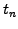, the scheme is said to be explicit. An implicit evaluation of the function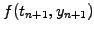is sometimes desirable in order to stabilize the propagation of numerical errors; this is however computationally expensive when the function cannot be inverted analytically.

More precision can be obtained by using a finite difference quotient symmetric in time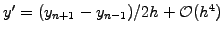, which is obtained by summing two Taylor series forward and backward (exercise 1.02). This yields the so-called midpoint formula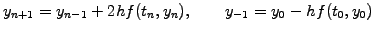(1.2.1#eq.3)

which is second order accurate, but requires a special initialization to generate the additional values that are needed from the past. Writing the mid-point rule as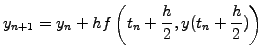the initialization problem is cured and leads to the famous second order Runge-Kutta method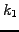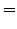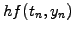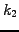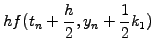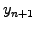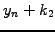(1.2.1#eq.4)

Such elementary and more sophisticated methods are commonly available in software packages such as OCTAVE and MATLAB; type help ode23 to find out more about how to use them. Methods for ordinary equations are usually robust; however, they become extremely inefficient when a problem is stiff, i.e. involves two very different scales by limiting the step size to the shorter scale even when this is not at all relevant (exercise 1.06).

SYLLABUS  Previous: 1.2 Differential Equations  Up: 1.2 Differential Equations  Next: 1.2.2 Partial differential equations